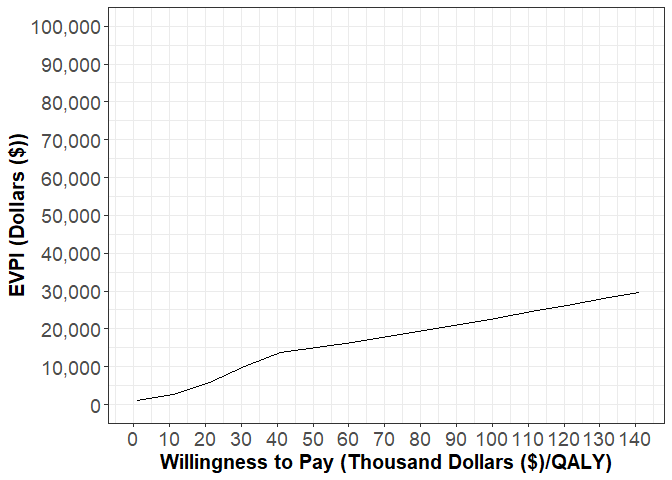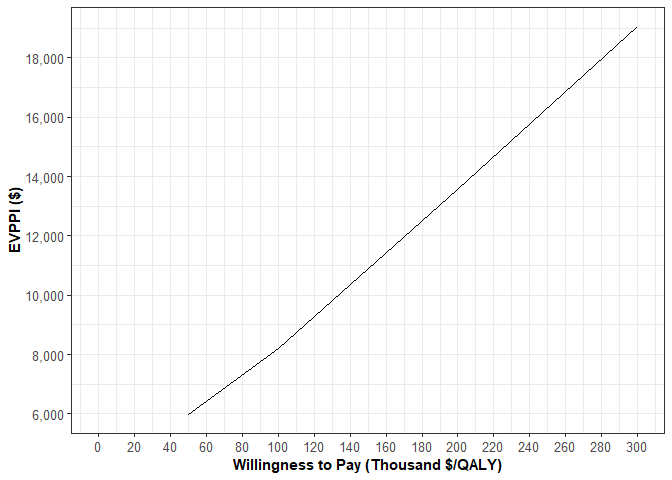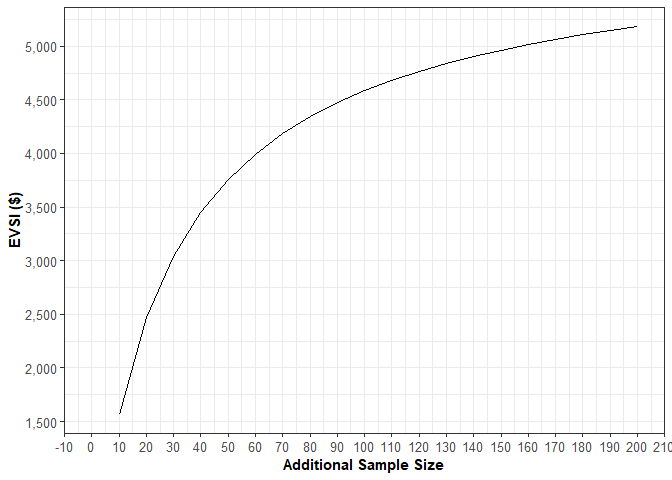# Overview

Value of information analysis (VoI) is a method for estimating the expected monetary gain from reducing uncertainty in the input parameters of a decision analytic model. Although a particular strategy is optimal because it maximizes expected net benefit given current information, this strategy could be suboptimal for certain plausible combinations of parameter values. Therefore, the greater the uncertainty in the model input parameters, the greater the risk that the decision that is optimal in expectation is actually suboptimal in reality. Parameter uncertainty can be reduced through new clinical trials and/or observational studies, and VoI analysis is a useful tool for guiding the optimal allocation of research funds. The decision to fund a future research effort is only advisable if the expected benefit of the resulting reduction in parameter uncertainty outweighs the cost of executing the research effort.

Although there are multiple methods for estimating value of information, the dampack package exclusively uses the probabilistic sensitivity analysis (PSA) for all of its VoI estimation functions. For more information about creation and analysis of PSAs in dampack, please refer to the vignettes for psa_generation and psa_analysis before proceeding.

# EVPI

The expected value of perfect information (EVPI) can be framed as the expected benefit of performing a study with an infinite sample size that would allow us to know the values of all the input parameters with complete certainty. With perfect information, there would be no uncertainty in the output of the decision model and the truly optimal strategy could be identified. In dampack, EVPI is calculated as the average opportunity loss across all samples in a probabilistic sensitivity analysis. The opportunity loss for each sample in the PSA is the difference between the expected benefit of the optimal strategy in that specific sample and the expected benefit of the strategy that would have been chosen on the basis of average expected benefit over the entire PSA. In other words, opportunity loss is the benefit that is foregone when making the optimal decision given imperfect information if the parameter values in that sample are correct.

In ‘dampack’, EVPI is calculated using the function calc_evpi(), which requires a psa object (see ?make_psa_object, ?gen_psa_samp, ?run_psa), and a vector of numeric willingness-to-pay (wtp) thresholds as function inputs. EVPI is frequently reported in terms of the net benefit of perfect information per patient, but calc_evpi also provides an option to calculate the total EVPI for an entire population. If the benefits in the psa object already reflect the aggregated benefits for the entire population of interest, leave the pop input at its default value of 1. calc_evpi() returns a data.frame of object class evpi containing the calculated EVPI at each WTP threshold provided in the input vector.

library(dampack)
data("example_psa")
psa_big <- make_psa_obj(example_psa$cost, example_psa$effectiveness,
example_psa$parameters, example_psa$strategies)
evpi_obj <- calc_evpi(psa = psa_big,
wtp = example_psa$wtp, pop = 1) head(evpi_obj) #> WTP EVPI #> 1 1000 1084.833 #> 2 11000 2613.132 #> 3 21000 5781.220 #> 4 31000 10023.211 #> 5 41000 13847.286 #> 6 51000 15086.647 The results contained in evpi_obj can be visualized using the plot() function, which has its own method for the evpi object class. p <- plot(evpi_obj, txtsize = 16, effect_units = "QALY", currency = "Dollars ($)",
xbreaks = seq(0, 200, by = 10),
ylim = c(0, 100000))
pFor a full listing of the options for customizing the EVPI plot, type ?plot.evpi in the console. Like all plots in dampack, the evpi plot object is a ggplot object, so we can add (+) any of the normal ggplot adjustments to the plot. A introduction to ggplot2 is hosted at https://ggplot2.tidyverse.org/.

# Metamodeling VoI Functions

dampack uses a technique known as metamodeling in its approach to estimating two other VoI measures, the expected value of partial perfect information (EVPPI) and the expected value of sample information (EVSI). In this context, a metamodel is a regression model that treats the expected loss of each strategy as the dependent variable and the decision model parameters of interest as the predictors. The expected losses for each row and strategy form a set of observations of the dependent variable, and the corresponding parameter values for those PSA samples form a set of predictor values for the regression.

The metamodeling functions are internal to dampack’s VoI functions, but they can also be manually called by the user for the diagnosis model fit, among other things. These metamodeling functions will be explored in greater detail at the end of this vignette.

# EVPPI

The expected value of partial pefect information (EVPPI) is the expected value of perfect information from only a subset of parameters of interest in a PSA. The function calc_evppi computes the EVPPI from a PSA using the following these steps:

1. The optimal strategy given current information is determined from the PSA.
2. The opportunity loss for each strategy is computed relative to the optimal strategy.
3. Using either generalized additive models (GAM) or polynomial models, the opportunity loss for each strategy across each PSA sample is regressed on basis functions of the parameters of interest. The GAM metamodel is fit using the ‘mgcv’ package.
4. For each strategy, the opportunity loss attributable to the parameters of interest is estimated by the fitted metamodel, and the expected benefit of completely removing the uncertainty in these parameters is calculated in a fashion analogous to calc_evpi
evppi <- calc_evppi(psa = psa_big,
wtp = c(5e4, 1e5, 2e5, 3e5),
params = c("pFailSurg", "pFailChemo"),
outcome = "nmb",
type = "gam",
k = 3,
pop = 1,
progress = FALSE)
#>     WTP     EVPPI
#> 1 5e+04  5996.643
#> 2 1e+05  8213.325
#> 3 2e+05 13559.326
#> 4 3e+05 19051.567

plot(evppi)# EVSI

Whereas EVPI and EVPPI considers the benefit of removing all uncertainty from all or some parameters, expected value of sample information (EVSI) considers the benefit of reducing only some uncertainty through a future study of finite sample size. In some sense, EVSI is more practical than EVPI and EVPPI because it is impossible to obtain absolutely perfect information about the model parameters. EVPI and EVPPI can give us an upper limit on how much money should be allocated to future research projects, but EVSI can provide an estimate for the return on investment for a financially realistic research design of effective sample size n. As the proposed effective sample size (n) approaches infinity, the EVSI approaches either the EVPPI or EVPI of the PSA, depending upon the parameters of interest.

dampack’s calculation of EVSI follows the same steps as the calculation for EVPPI until the point at which the metamodel is used to estimate the opportunity loss of each strategy for each PSA sample. Assuming that the means and variances of the underlying parameter distributions in the PSA came from a previous study of effective sample size n0, a Gaussian approximation is used to estimate the new means and variances of these parameters after a hypothetical study of effective sample size n has been completed. For in-depth details on dampack’s methodology for estimating EVPPI and EVSI, please refer to:

Jalal H, Alarid-Escudero F. A General Gaussian Approximation Approach for Value of Information Analysis. Med Decis Making. 2018;38(2):174-188.

evsi <- calc_evsi(psa = psa_big,
wtp = 5e4,
params = c("pFailSurg", "pFailChemo"),
outcome = "nmb",
k = 3,
n = seq(from = 10, to = 200, by = 10),
n0 = 50,
pop = 1,
progress = FALSE)

#>     WTP  n     EVSI
#> 1 50000 10 1569.751
#> 2 50000 20 2468.848
#> 3 50000 30 3042.956
#> 4 50000 40 3448.690
#> 5 50000 50 3753.183
#> 6 50000 60 3991.067

plot(evsi)By default, calc_evsi will assume that all parameters included in params came from the same study of sample size n0 and will both be informed by a new study of sample size n. Providing a sequence of values for n will allow the user to assess how EVSI changes as a function of the additional sample size. However, it may be the case that each parameter in params came from a separate study with a unique initial sample size n0, and a unique additional sample size n. If this is the case, the user should set argument n_by_param to TRUE, and provide a numeric vector of sample sizes for n0 and n corresponding to the order of the parameters in params.

# Metamodeling Appendix

In essence, the metamodel function takes a PSA object and creates a regression model for each strategy specified that predicts the outcome using the parameters specified in the params argument. These regression models are stored in a nested list within the metamodel object, where they can be accessed to diagnose model fit, predict outcomes conditional on specific parameter values, and perform any other supported functions allowed by the model type.

The simplest type of metamodel that can be fit is an ordinary linear regression model via lm, which can be specified by setting type = "linear". Linear models fit by metamodel utilize the outcome specified as the dependent variable (see ?calculate_outcomes), and contain terms only for the parameters in params without interaction terms. Although linear models are useful for their interpretability and simplicity, they do not adequately capture the nonlinear relationships that often arise in mathematical models.

More flexible polynomial regression models can be fit by setting type = "poly" and specifying the maximum polynomial degree with the poly.order argument. This model type is also fit via the lm function, but rather than predicting the outcome based on only the first degree term for each parameter in the params argument, a separate term is included for each parameter from the first degree up to and including the degree specified by the poly.order argument. Using a poly.order that is too high can lead to overfitting.

Although both first degree and higher order linear regression models are capable of utilizing interaction terms, the formulas for these model types in dampack do not contain interaction terms, except when called within the context of a two-way sensitivity analysis. When a few influential parameters exhibit significant interaction and/or nonlinear effects, it is recommended that one fit the metamodel with a generalized additive model by setting type = "gam". The generalized additive model (GAM) is fit through the gam() function of the package mgcv, and in-depth advice on customizing the models, diagnosing model fit, and interpreting the results can be found in the help documentation for that package. In short, generalized additive models are generalized linear models in which the linear predictor depends linearly on smooth functions (i.e. penalized regression splines) of some predictor variables. Interactions between the parameters in params are fit using tensor product interaction smooths (see ?mgcv::ti). A smooth term of one or several variables is the sum of some number of basis functions that combine to flexibly fit the data. The number of basis functions used to fit the GAM model in metamodel() is pulled from the argument k, and the higher the number of basis functions, the more flexible the resulting GAM model. GAM models are susceptible to overfitting, and care should be taken to choose the minimum k value that adequately captures any nonlinear main effects and interactions.

According to the mgcv help documentation, “k should be chosen to be large enough that you are reasonably sure of having enough degrees of freedom to represent the underlying ‘truth’ reasonably well, but small enough to maintain reasonable computational efficiency. Clearly ‘large’ and ‘small’ are dependent on the particular problem being addressed.” dampack users should note that trying to fit GAM metamodels (either through metamodel() directly or through calc_evsi() or calc_evppi) with several parameters and high values of k will frequently lead to errors in the gam function of mgcv. For multiway GAM metamodels, if the supplied k value is below 3 then it will automatically set to 3. If a k value lower than 3 is used, mgcv will automatically choose a higher k value that will frequently cause the model to fit slowly and/or cause R to crash. When type = "gam", k-1 to the power of the number of parameters must not be greater than 500 (i.e. (k-1)^length(params) < 500). Empirically, models that exceed this constraint will either take an excessively long time to run or simply cause R to crash.

mm <- metamodel(analysis = "twoway",
psa = psa_big,
params = c("pFailChemo", "cChemo"),
strategies = "Chemo",
outcome = "eff",
type = "gam")
#> Warning in mm_run_reg(analysis, s, params, dat, type, poly.order, k): k has been
#> set to its minimum value of 3

The objects produced by the metamodel() have specialized print, summary, and predict methods.

The output of the metamodel function can be inspected using the print function. Some key pieces of information include 1) the outcome that was modeled as the dependent variable, 2) the willingness-to-pay threshold that was assumed (only relevant for net health benefit and net monetary benefit), 3) the strategies for which a metamodel was produced, and 4) the set of PSA parameters that were modeled as the independent variables in the regressions.

print(mm)
#> metamodel object
#> -------------------------
#> a list of the following objects: outcome, mods, wtp, params, strategies, psa, analysis, type, poly.order, k
#>
#> some details:
#> -------------------------
#> analysis: this is a two-way metamodel
#> mods: a nested list of gam metamodels
#> outcome: eff
#> WTP: NA
#> strategies: Chemo
#> parameters modeled: pFailChemo, cChemo

Using the summary function on a metamodel object produces a data.frame with a row for each regression model in the nested list within the metamodel object. The first column for a one-way metamodel and the first two columns for a two-way metamodel show the PSA parameter(s) treated as the independent variable(s) in the regression, and the “strat” column shows the strategy for which regression model was fit. A separate regression model can be fit for each specified strategy, and models are fit for all strategies within the calc_evppi and calc_evsi functions. The final value is the adjusted r-squared for the model, which is defined as the proportion of variance in the outcome variable explained by variance in the parameter(s) included in the model. This adjusted r-squared value can be thought of as a measure of how influential the selected parameter(s) is/are in affecting the model outcome. A low adjusted r-squared value does not necessarily mean that the metamodel is a bad statistical model, but rather that the parameters considered only explain a small fraction of the variation in the outcome. A negative adjusted r-squared value implies that the regression model explained less variance than the model with only an intercept term.

summary(mm)
#>       param1 param2 strat   rsquared
#> 1 pFailChemo cChemo Chemo 0.04426551

In general, the predict function will create a data.frame of parameter values based off of user input and the supplied metamodel, and use the metamodel to predict outcome values over every combination of input values in the data.frame. predict() has slightly differing functionality depending on whether or not the metamodel object supplied was produced with analysis = "oneway" or analysis = "twoway". The ranges argument must be a named list that gives the ranges for the parameters of interest, and the nsamp argument must be an integer. The nsamp argument tells the function how many evenly spaced values between the ranges of each parameter should be drawn in constructing the data.frame of parameter inputs. For one-way metamodels, the vector of parameter values dictated by ranges and nsamp will be sequentially fed into the corresponding regression model to yield a predicted output. This process is performed separately for each parameter named in the ranges argument. For two-way metamodels, the same general process is applied, except that an nsamp by nsamp grid of the two relevant parameter inputs is ultimately fed into the regression model. For one-way analyses, these regression models only use the parameter of interest as the independent variable and therefore it is not necessary to supply the other parameter values. For the two-way analyses, both parameters of interest are incorporated as covariates in the regression model, and values for both must be supplied to produce outcome predictions.

If a parameter name is supplied in the ranges argument but the vector of ranges itself is left as NULL, then the 2.5th and 97.5th quantiles of the parameter values from the PSA will be automatically supplied as the range.

pred_mm <- predict(mm,
ranges = list("pFailChemo" = c(0.3, 0.6),
"cChemo" = NULL),
nsamp = 10)
#> 6  0.4666667 16291.4    Chemo    10.95724